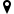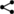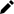Welcome guest
You're not logged in.
313 users online, thereof 0 logged in

## Discrete Calculus and Difference Equations

This part is dedicated to the so-called discrete calculus, sometimes also called the calculus of finite differences. This is a subject providing methods for solving difference equations. As opposed to differential equations, difference equations are useful as a mathematical representation for certain physical problems.

The purpose of this part is to develop a calculus of “differences” and a “sums”, in analogy to the calculus of derivatives and Riemann integrals.|||| created: 2020-03-23 20:38:13 | modified: 2020-03-25 19:23:22 | by: bookofproofs | references: , ,